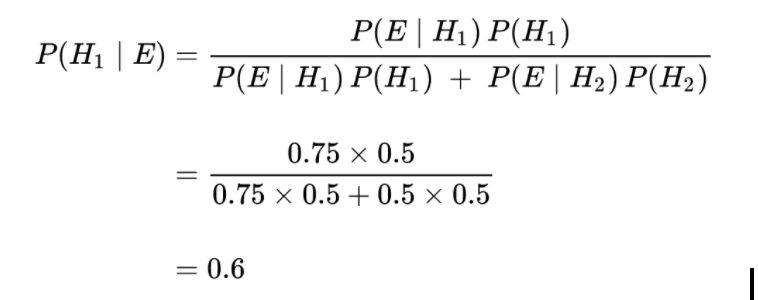# Bayesian Inference

## What is Bayesian Inference?

Bayesian inference refers to the application of Bayes’ Theorem in determining the updated probability of a hypothesis given new information. Bayesian inference allows the posterior probability (updated probability considering new evidence) to be calculated given the prior probability of a hypothesis and a likelihood function.

## Example of Bayesian inference

Bayesian inference is probably best explained through a practical example. Let’s say that our friend Bob is selecting one marble from two bowls of marbles. The first bowl has 75 red marbles and 25 blue marbles. The second bowl has 50 red marbles and 50 blue marbles. Given that Bob is equally likely to choose from either bowl and does not discriminate between the marbles themselves, Bob in fact chooses a red marble. What is the probability Bob picked the marble from bowl #1?

Let’s call the possibility that Bob chose a marble from bowl #1 H1 (Hypothesis 1) and the possibility he chose a marble from bowl #2 H2 (Hypothesis 2).

If we know that Bob believes the bowls are identical, then the probability of hypothesis 1 is equal to the probability of hypothesis 2 ( P(H1) = P(H2) ), and both hypotheses must be equal to one (the total probability), making them each 0.5.

Now we’ll call the observation of a blue marble, event E. Given the distribution of the marbles in each bowl, we know that :

P(E|H1) = 75/100 = 0.75

P(E|H2) = 50/100 = 0.50

Plugging in these probabilities into Bayes’ formula we get:To recap, before we used the observational data from Bob’s choice, the probability that he chose a marble from bowl #1 (Hypothesis 1) was 0.5 because the bowls were equal from Bob’s point of view.  After we observe that he chose a red marble, and apply Bayes’ theorem, we revise the probability of Hypothesis 1 to 0.6. This is Bayesian inference, using new information to update a probabilistic model.